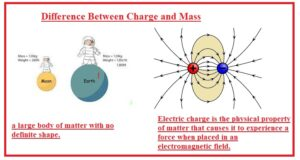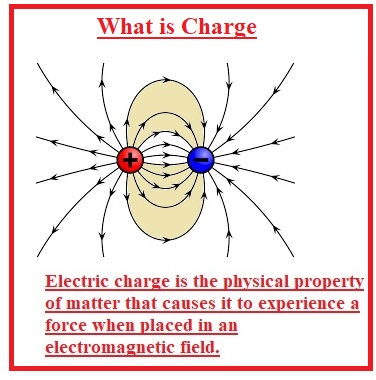Hello, readers welcome to new post. Here we will discuss Difference Between Charge and Mass. Charge and mass are considered as the properties of matter. The basic difference between these two properties are that charge has types and mass does not have any types. The charge can be positive and negative and mass is only positive.

In this post, we will discuss definition uses and other features with differences between these two terms. SO let’s get started

### Difference Between Charge and Mass

What is Mass

• Mass is matter quantity in any object or body. Mass also called the measurement of inertia.
• it is also called acceleration if force is applied on the object.
• Mass of anybody also tells about the gravitation attraction for other bodies.
• System international unit of mass is kilogram and denoted as Kg.
• In case of physics, mass is not considered like weight.

Unit of Mass

• System international of unit of mass is KG. one Kg has one thousand grams. One kg is the mass of one cubic decimeter of water in case of melting ice.### What is Charge

• A charge is a physical feature of matter that results to bear a force on the object when it places in an electromagnetic field.
• The charge can be positive or negative on the basis of protons and electrons.
• Same charges repel each other and opposites attract one another. The object that has zero charges is called neutral.
• Charge has a constant value the total charge of isolated system the quantity of positive charge and minus quantity of negative charge has same value.
• Charges has subatomic particles negative charges ahs electrons and positive charges ahs protons.
• If an object has larger protons then has a positive charge and if have electrons than has a negative charge.
• Charges make an electric field and moving charges has a magnetic field. The combination of charges with an electromagnetic field results in an electromagnetic force that is considered a fundamental force.
• The System international unit of charge is coulomb named after its inventor. For electrical engineering unit of charge is the Faraday constant which is charge of one mole of electrons.
• A charge is denoted as q.### What is the relation between charge and mass?

• In come with charge to mass ratio is used which is the multiplicative inverse of the mass to charge ratio. According to CODATA value of electron is Qm = −1.75882001076(53)×1011 C⋅kg−1.

### Is mass equal to charge?

• Mass of electrons is 9.10938356 × 10-31 kilograms.
• Charge of electrons is 1.602 x 10-19 coulombs.

That is all about the Difference Between Charge and Mass all details has been explained if you have any further query ask in comments. Thanks for reading have a nice day